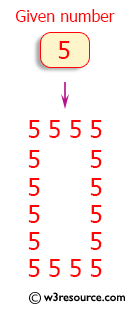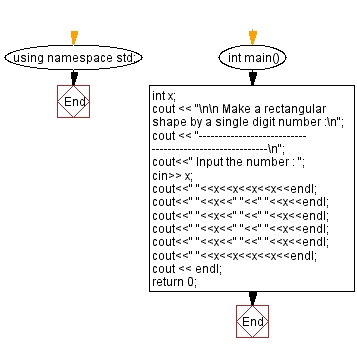﻿ C++ : Create a rectangle from a single digit number

# C++ Exercises: Print a single digit number and print a rectangular form of 4 columns and 6 rows

## C++ Basic: Exercise-31 with Solution

Write a C++ program to input a single-digit number and print it in a rectangular form of 4 columns and 6 rows.

Pictorial Presentation:Sample Solution:

C++ Code :

``````#include <iostream>
using namespace std;

int main()
{
int x;
cout << "\n\n Make a rectangular shape by a single digit number :\n";
cout << "--------------------------------------------------------\n";
cout<<" Input the number : ";
cin>> x;
cout<<" "<<x<<x<<x<<x<<endl;
cout<<" "<<x<<" "<<" "<<x<<endl;
cout<<" "<<x<<" "<<" "<<x<<endl;
cout<<" "<<x<<" "<<" "<<x<<endl;
cout<<" "<<x<<" "<<" "<<x<<endl;
cout<<" "<<x<<x<<x<<x<<endl;
cout << endl;
return 0;
}
``````

Sample Output:

``` Make a rectangular shape by a single digit number :
--------------------------------------------------------
Input the number : 5
5555
5  5
5  5
5  5
5  5
5555
```

Flowchart:C++ Code Editor:

What is the difficulty level of this exercise?

﻿

## C++ Programming: Tips of the Day

Why is there no std::stou?

The most pat answer would be that the C library has no corresponding "strtou", and the C++11 string functions are all just thinly veiled wrappers around the C library functions: The std::sto* functions mirror strto*, and the std::to_string functions use sprintf.

Ref: https://bit.ly/3wtz2qA

We are closing our Disqus commenting system for some maintenanace issues. You may write to us at reach[at]yahoo[dot]com or visit us at Facebook